Journal of Vibroengineering

Published: 15 May 2022

# Strength and vibration analysis of transonic compressor under multiple field loads

Yu Ru Feng1
Jun Li Wang2
Jin Yang Li3
1, 2, 3School of Mechanical Engineering, Shaanxi University of Technology, Hanzhong, 723000, China
2Shaanxi Key Laboratory of Industrial Automation, Shaanxi University of Technology, Hanzhong, 723000, China
Corresponding Author:
Jun Li Wang
Views 71

#### Abstract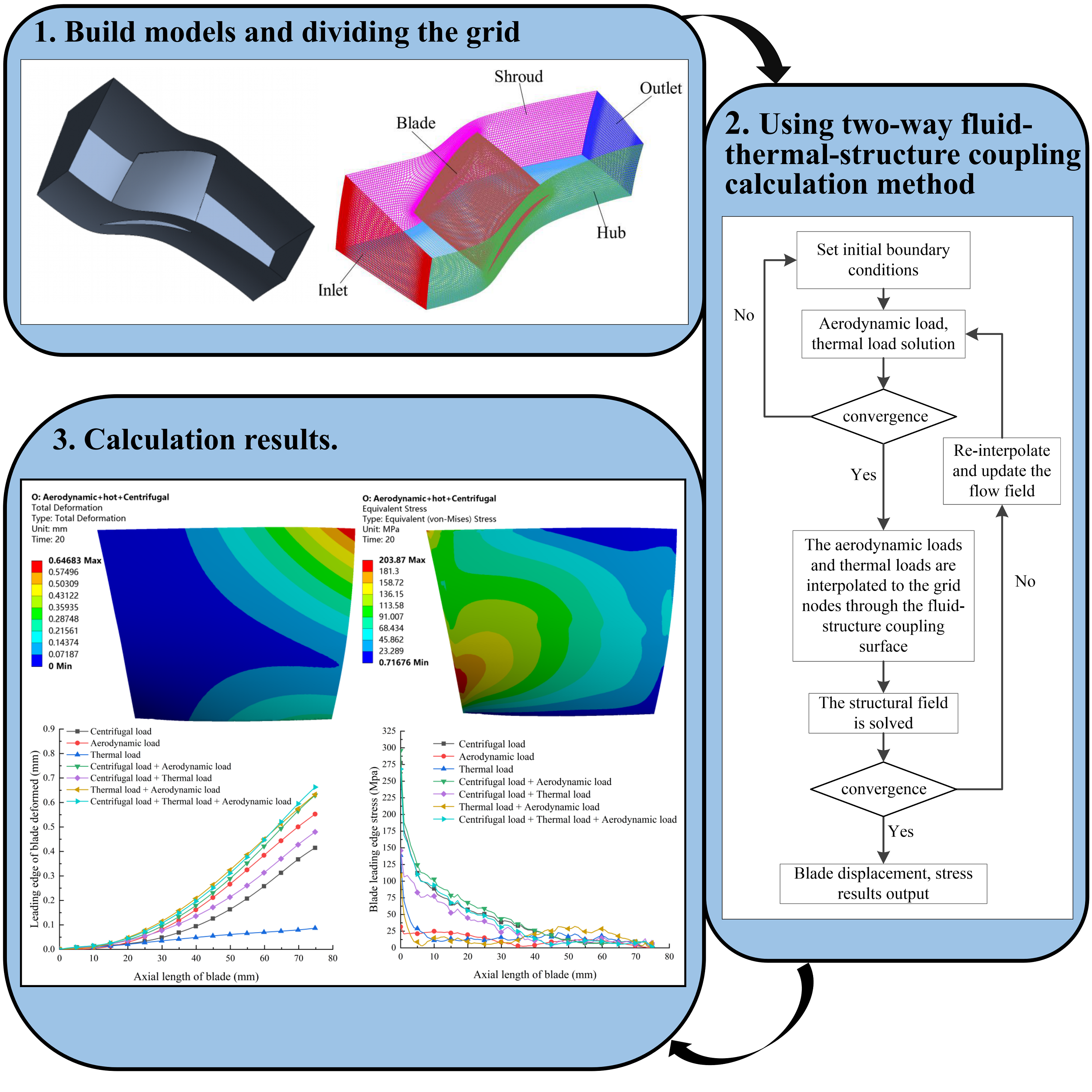#### Highlights

• The maximum deformation of the blade occurs at the tip of the leading edge. The stress of the blade is mainly concentrated at 33% of the position of the root of the blade.
• At different speeds, the deformation of the blades will first increase and then decrease with the continuous increase of the mass flow. The equivalent stress of the blades changes with the change of the mass flow and is related to the change of the speed.
• In the case of fluid-thermal-structure coupling, the natural frequency of the blade has a great change compared with the natural frequency, and the first-order vibration frequency with the biggest change increases by 25.77%.
• The compressor blades have a resonance point at about 63% and 98% speed.

## 1. Introduction

Compressor blade is one of the key parts of the engine, its safety and stability directly determine the performance of the engine. With the continuous improvement of aero-engine performance, the design trend of compressor tends to be higher pressure ratio and efficiency. However, high-speed rotating compressor blades bear large thermal stress, centrifugal force, aerodynamic force and other loads. According to the relevant data , compressor blade fracture accounted for more than 40 % of engine fault , compressor vibration fault accounted for more than 60 % of engine fault , compressor blade fault accounted for more than 70 % of vibration fault. Therefore, in order to simulate the real force of the compressor, it is necessary to comprehensively consider the influence of thermal stress, aerodynamic force and centrifugal force on the structural characteristics of the compressor, so as to obtain the force of the compressor in the real working environment.

In recent years, scholars in China and abroad have conducted a large number of studies on the compressor. In the study of the compressor flow field, Yamada and Funazaki  took NASA’s Rotor37 as the research object to study the interaction between the shock wave triggered by the supersonic blade and the tip leakage vortex in the tip clearance. Under the condition of standard clearance, the tip leakage vortex downstream of the shock wave breaks down under the condition of near stall. The breakdown of tip leakage vortex leads to the flow of low energy fluid downstream of shock wave in the rotor channel, resulting in a large blockage effect near the rotor tip. Zhang Yanfeng et al.  revealed the tip leakage characteristics of rotor Rotor 37 under near stall condition through steady and unsteady numerical simulation of Rotor 37, and further understood the influence of gap leakage flow on rotor stall.

In the aspect of fluid-structure coupling of compressor, Lee Horim et al.  obtained the influence of blade tip and impeller deformation on the performance of centrifugal compressor by fluid-structure coupling analysis of centrifugal compressor. Kovacevic et al.  used the finite element method to simulate the fluid-structure coupling of the screw compressor. The results show that the compressor will produce huge pressure difference and temperature difference on the rotor during the compression process, so that the impeller blade will be deformed. The deformation will cause the tip clearance to change, which will seriously affect the performance of the compressor. Therefore, it is necessary to carry out fluid-structure coupling simulation to study this. Tao Hailiang et al.  used the fluid-structure coupling method to carry out the numerical simulation of the aeroelasticity of the compressor blade, and used the unsteady fluid-structure coupling method to obtain the dynamic stress and dynamic strain analysis of the blade caused by the aerodynamic shock, so as to determine whether the compressor blade will have flutter, which provides a theoretical basis for the subsequent strength analysis. Du Zixue et al.  studied the influence of fluid-structure coupling field on blade strength and vibration parameters. The results show that the fluid-structure coupling has little effect on the structural strength and modal shape of the blade. Through frequency analysis, the resonant frequency of the blade is found out, which provides a basis for the optimization of the blade.

In the research of compressor temperature field, Mustafin T. N. et al.  designed and proposed a numerical simulation model to calculate the rotor temperature field under working condition, and analyzed the influence of structural parameters and working conditions of screw compressor on rotor temperature field. Based on fluid-thermal-structure coupling coupling, the strength analysis of a new type of gas turbine transonic compressor impeller under comprehensive load was carried out by Li Guangxin et al. .It is concluded that the maximum deformation of the impeller occurs at the tip of the leading edge of the splitter blade, and the maximum stress occurs at the chamfer of the back hole of the hub, which provides an engineering design calculation method for the strength analysis of the impeller and the reliable design of the compressor. Li Chunwang et al.  found a modal analysis method of engine blade with thermal-structure coupling prestress considering the combined effect of thermal load, centrifugal load and aerodynamic load. Wang Dingbiao et al.  took a centrifugal compressor impeller as the research object, and obtained the stress distribution and maximum deformation of the blade under the combined action of centrifugal force, aerodynamic force and thermal load.

## 2. Calculation method

Fig. 1Fluid-thermal-structure coupling process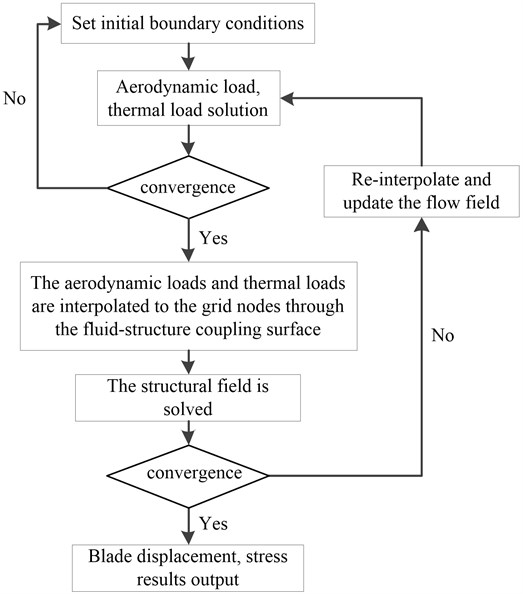## 3. Model establishment

### 3.1.1. Governing equations of fluids

Fluid flow must follow the laws of conservation of physics. The basic laws of conservation include the law of conservation of mass, the law of conservation of momentum, and the law of conservation of energy . If the fluid includes other mixed components, the system must also follow the law of conservation of components. For the general compressible Newtonian flow, the conservation law is described by the following governing equation.

Mass conservation equation:

1
$\frac{\partial {\rho }_{\mathrm{f}}}{\partial t}=\nabla \cdot \left({\rho }_{f}v\right)=0.$

Momentum conservation equation:

2
$\frac{\partial {\rho }_{f}}{\partial t}=\nabla \cdot \left({\rho }_{f}vv-{\tau }_{f}\right)={f}_{f}.$

Among them, $t$ represents time and ${f}_{f}$ is the volumetric force vector. ${p}_{f}$ is the fluid density, $v$ is the fluid velocity vector, and ${\tau }_{f}$ is the shear force tensor, which can be expressed as Eq. (3):

3
${\tau }_{f}=\left(-p+u\nabla \cdot v\right)I+2ue,$

where, $p$ is fluid pressure, $u$ is dynamic viscosity, $e$ is velocity stress tensor:

4
$e=\frac{1}{2}\left(\nabla v+\nabla {v}^{T}\right).$

### 3.1.2. Governing equations of structures

For the solid field is mainly the deformation of the solid caused by the action of the fluid force in the fluid field, the conservation equation of the solid part can be derived from Newton’s second law:

5
${\rho }_{\mathrm{s}}{\stackrel{¨}{d}}_{s}=\nabla \cdot {\sigma }_{s}+{f}_{s},$

where ${p}_{s}$ is the density of structures, ${\sigma }_{s}$ is the Cauchy stress tensor, and ${f}_{s}$ is the volume force vector. ${\stackrel{¨}{d}}_{s}$ is the local acceleration vector in the structure domain:

6
$\frac{\partial \left(\rho {h}_{tot}\right)}{\partial t}-\frac{\partial p}{\partial t}+\nabla \cdot \left({\rho }_{f}v{h}_{tot}\right)=\nabla \cdot \left(\lambda \nabla T\right)+\nabla \cdot \left(v\cdot \tau \right)+v\cdot \rho {f}_{f}+{S}_{E},$

where, $\lambda$ represents the thermal conductivity, and ${S}_{E}$ represents the energy source term.

For the structure part, the thermal deformation term caused by temperature difference is added. Its mathematical expression is as shown in Eq. (7):

7
${f}_{T}={\alpha }_{T}\cdot \nabla T,$

where ${\alpha }_{T}$ is the thermal expansion coefficient dependent on temperature.

### 3.1.3. Fluid-structure interaction coupling equation

Fluid-structure coupling follows the most basic principle of conservation, so it is at the interface of fluid-structure coupling. Should satisfy the fluid and solid stress ($\tau$), displacement ($d$), heat flow ($q$), temperature ($T$) and other variables are equal , that is, Eq. (8) is satisfied:

8
$\left\{\begin{array}{l}{\tau }_{f}\cdot {n}_{f}={\tau }_{s}\cdot {n}_{s},\\ {d}_{f}={d}_{s},\\ {q}_{f}={q}_{s},\\ {T}_{f}={T}_{s}.\end{array}\right\$

In Eq. (8), subscript $f$ stands for fluid, and the subscript $s$ stands for solid.

### 3.1.4. Thermal-structure coupling equation

The temperature field and thermoelastic finite element equation of the thermosetting unidirectional calculation model are as follows:

9
$M\frac{\partial T}{\partial t}+KT-Q=0,$
10
$DU=GT+F.$

In Eq. (9) and Eq. (10), $M$ is the heat capacity matrix; $T$ is the temperature vector; $t$ is the time; $K$ is the heat conduction matrix; $Q$ is the heat flow vector; $D$ is the stiffness matrix; $U$ is the displacement vector; $G$ is the thermal stress coefficient matrix; $F$ is the mechanical force vector.

### 3.1.5. Basic theory of compressor blade modal analysis

Modal analysis is a technique used to determine the vibration characteristics of a structure. The main application is to establish a predictive model of the dynamic response of the structure to serve the dynamic strength design of the structure. For a multi-degree-of-freedom vibration system, the dynamics of the elastic structure can be solved according to the D'Alembert principle to derive the dynamic balance equation :

11
$\left[M\right]\left\{\stackrel{¨}{u}\right\}+\left[C\right]\left\{\stackrel{˙}{u}\right\}+\left[K\right]\left\{u\right\}=\left\{F\left(t\right)\right\},$

where: $\left[M\right]$ is the mass matrix; $\left[C\right]$ is the damping matrix; $\left[K\right]$ is the stiffness matrix; $\left\{\stackrel{¨}{u}\right\}$ is the acceleration vector; $\left\{\stackrel{˙}{u}\right\}$ is the velocity vector, $u$ is the displacement vector; $F\left(t\right)$ is the force vector.

### 3.2. Physical model

The research object of this paper is the NASA rotor Rotor37, which was originally designed and tested by Reid and Moore of Glenn Research Center in the United States. The design parameters and experimental data can be found in reference . Rotor37 is the rotor in Stage37 of a four-stage axial compressor with a high boost ratio (20:1). The geometric parameters are shown in Table 1. Fig. 2 is a schematic diagram of the single-channel model of the rotor.

Table 1Basic design geometrical parameters of rotor

 Parameter Design value Total rotor pressure ratio 2.106 Total rotor temperature ratio 1.261 The adiabatic efficiency 0.877 Apical clearance / mm 0.356 Design mass flow / (kg∙s-1) 20.188 Design speed / (r∙min-1) 17188.7 Leaf number 36

The research object of this paper is the NASA rotor Rotor 37, which was originally designed and tested by Reid and Moore from Glenn Research Center in the United States. The design parameters and experimental data can be found in the reference. Rotor 37 is a rotor in a four-stage axial compressor Stage 37 with a high pressurization ratio of 20:1. The geometrical parameters are shown in Table 1. Fig. 2 is a schematic diagram of the rotor mode, Fig. 2(a) is a full-channel model, Fig. 2(b) is a single-channel model.

Fig. 2Sketch of Rotor 37 model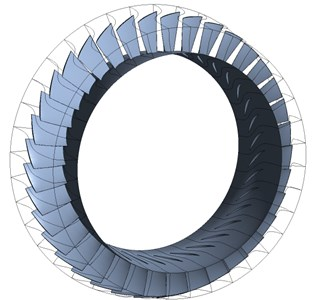a) Pressure ratio characteristic curve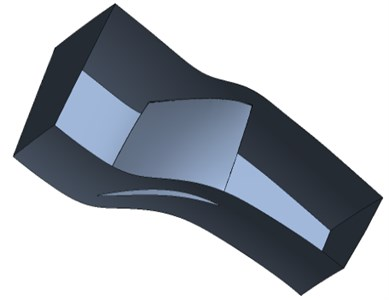b) Efficiency characteristic curve

### 3.3. Meshing and independence verification

This paper uses the TurboGrid software in ANSYS Workbench to divide the grid. When dividing the grid, all the inlet and outlet sections of the calculation domain are extended to the outer diameter of the casing to prevent the reflected pressure wave at the inlet and outlet boundaries from generating the internal flow of the compressor. Influence. In this paper, the rotor blade channel adopts H-O-H structured grid, the blade adopts O-shaped grid, the inlet and outlet adopt H-shaped grid, and the gap adopts a combined butterfly-shaped and H-shaped grid structure. Increase the number of meshes near the wall and the tip of the blade to refine the mesh.

The number of grids directly affects the accuracy of the numerical simulation. Too many grids will increase the amount of calculation, and too few grids will cause the calculation results to be distorted. In order to verify whether the grid density is reasonable, five sets of grids are drawn separately. In order to save computing resources, according to the research results of the literature , grids with 258000, 456000, 741200, 1.068 million, and 1.56 million nodes were selected to verify the grid independence of the flow field. It can be seen from Fig. 3 that as the number of grids increases, the isentropic efficiency gradually increases, but when the number of grids reaches a certain value, the isentropic efficiency does not change with the increase of the number of grids. It can be seen from Fig. 3 that when the number of nodes is greater than 741200, the isentropic efficiency does not show a significant change. Table 2 shows the comparison between the isentropic efficiency obtained by numerical simulation and the experimental value under different grid numbers. It can be seen from Table 2 that when the number of nodes is greater than 741200, the isentropic efficiency does not change significantly with the increase of the grid, and the error of isentropic efficiency with the experimental design point is 2.3 %. Therefore, it can be considered that the number of nodes has reached the requirement of grid independence. For reasonable calculation accuracy and calculation speed, this paper selects a grid with a total number of 741200 for the numerical calculation of the blade flow field. Fig. 4 shows the runner grid.

Table 2Comparison of numerical simulation results with experimental values

 Grid number Experiment / % Simulation / % Error / % 25.8w 87.7 84.35 3.82 45.6w 87.7 84.65 3.47 74.12w 87.7 85.68 2.30 106.8w 87.7 85.61 2.38 150w 87.7 85.72 2.25

Fig. 3Grid independence verification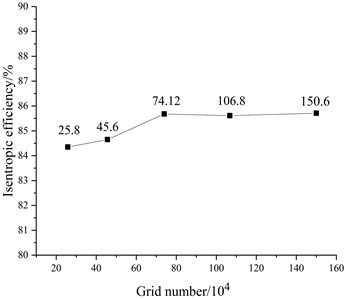Fig. 4Port grid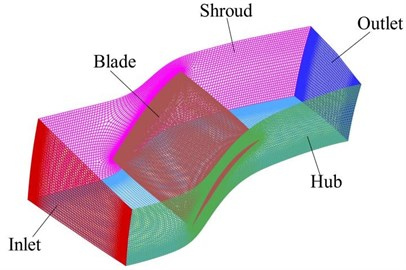### 3.4. Moving mesh model

In the process of fluid-thermal-structure coupling solution, the deformation of the structure motion will change the flow field. Therefore, in the process of numerical simulation, the CFD grid must change with the deformation of the structure. In the iterative process of calculation at each time step, the finite volume grid in the CFD calculation domain must follow the structure to adapt to a new position and be solved in a new state. The dynamic grid is the entire process of solving at each time step, the blade vibration displacement causes the shape of the flow field to change, and the CFD grid must follow the structure to adapt to the new position. In this paper, we use CFX software to realize the movement of the grid, that is, the dynamic grid. In the calculation process, the grid node displacement changes on the coupled interface are obtained by interpolation of the blade surface vibration displacement. At the same time, in order to avoid serious grid deformation, the grid quality will be reduced and the calculation static will be affected. The flow field grid nodes around the blade are also Displacement must be generated accordingly. Before each flow field is iteratively calculated, the node displacement change is obtained by solving the mesh displacement equation, and then the coordinate value of the mesh node is updated to form a new flow field grid.

### 3.5. Solve settings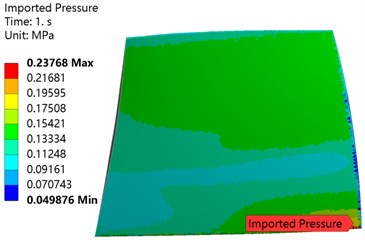a) Pressure surface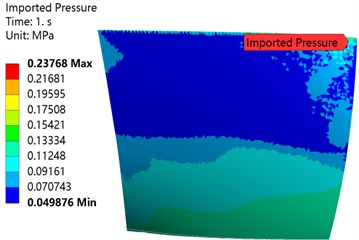b) Suction surface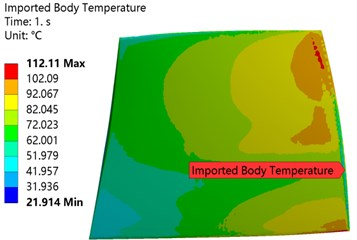a) Pressure surface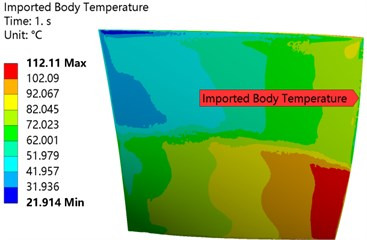b) Suction surface

### 3.6. Model validation

Combining experiments and classic literature to verify the accuracy of the results, Fig. 7 shows the compressor characteristic curve. After calculation, the design point pressure ratio of the numerical calculation has an error of 3.84 % compared with the experimental value .

Fig. 7Comparison of calculated results and experimental results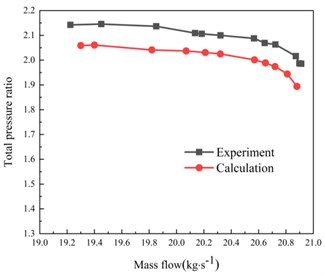a) Pressure ratio characteristic curve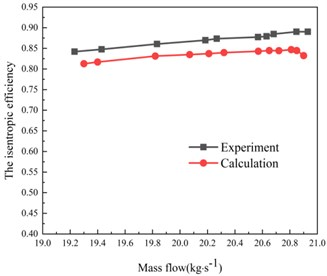b) Efficiency characteristic curve

The value of the isentropic efficiency is compared with the experimental value. The error is 2.3 %. However, the variation trend of numerical calculation and experimental results is basically the same, and the error is also within the acceptable range. The error may be related to the selected turbulence model and insufficient accuracy of the Rotor37 data. According to the experimental data in literature , at the design speed, the clogging flow of the rotor is 20.93 kg/s, and the calculated value in this paper is 20.9 kg/s, the difference between the two is 0.14 %. In addition, the stall of the rotor the flow rate is 19.36 kg/s, the calculated value predicted in this article is 19.3 kg/s, the difference between the two is 0.30 %. It can be seen that the calculation method in this paper can predict the stall boundary and blockage boundary of the rotor more accurately.

## 4. Result analysis

In order to understand the effect of different loads on the deformation and stress of the blade more clearly, this paper analyzes based on the following situations, 1) only consider the blade’s aerodynamic load on the flow field, 2) only consider the blade’s centrifugal load, 3) only consider the blade heat Load effect; 4) consider the coupling effect of different loads.

 The load The biggest deformation (mm) Maximum equivalent stress (MPa) Only subjected to aerodynamic load 0.58938 57.16 Only under thermal load 0.060304 258.67 Centrifugal loads only 0.41516 277.41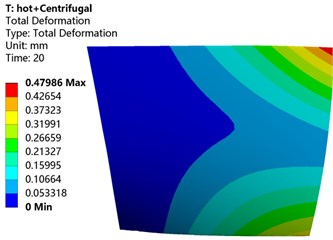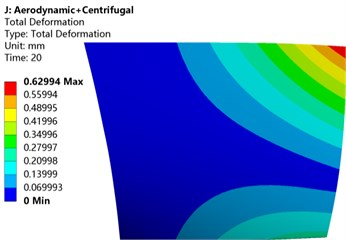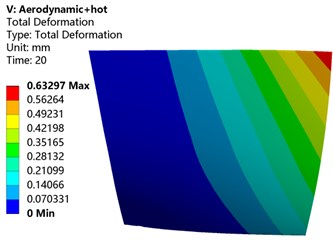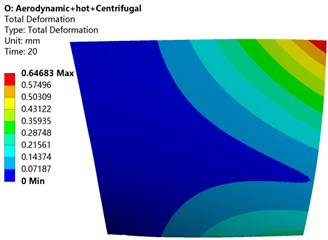Fig. 9Variations of blade deformation along the path of the leading edge under coupling of different loads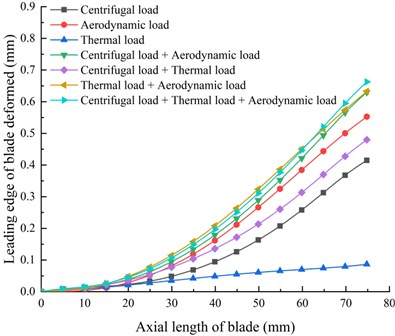Fig. 10Variation of blade deformation with mass flow at different speeds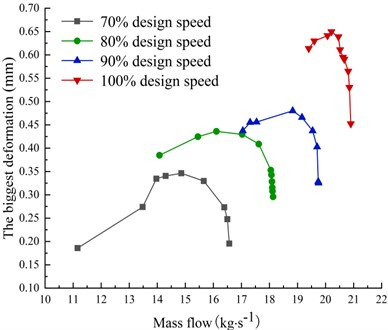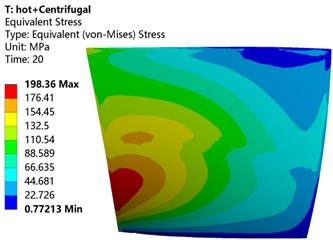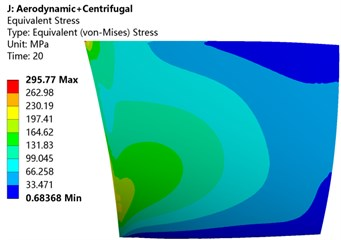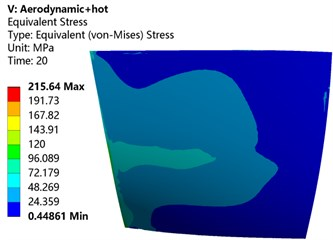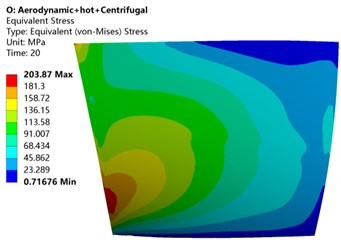Fig. 12Variation of blade equivalent stress along the path of the leading edge under different load coupling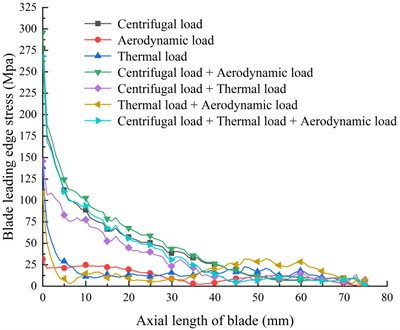It can be seen from the above analysis that when we analyze and check the strength of the blade, we must take the thermal load into account. That is, we must consider the combined effect of thermal load, centrifugal load and aerodynamic load to be able to simulate the working conditions of the blade to the greatest extent.

Fig. 13Variation of blade equivalent stress with mass flow at different speeds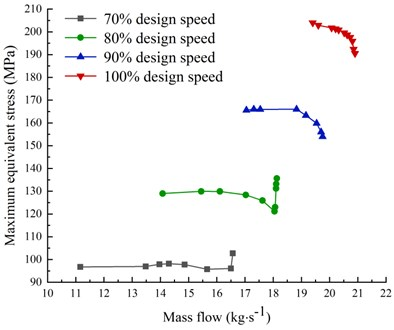### 4.3. Modal analysis and resonance judgment of blades under different prestresses

For rotating machinery, if the natural frequency of the blade is the same as or close to the excitation frequency generated by the system during rotation, it will cause the blade to resonate, produce larger amplitude and vibration stress, and easily cause high-cycle or low-cycle fatigue fracture. Campbell diagram can determine whether the frequency of the various excitation forces generated by the rotating parts of the compressor within the operating speed range of the compressor is consistent with the natural frequency of some parts , , which can effectively prevent the occurrence of resonance and damage to the parts. Based on the thermal load, aerodynamic load and centrifugal load prestress, the modal analysis of the blades at different speeds is carried out, and the natural frequencies of the blades at different speeds are obtained as shown in the Table 5. Through the natural frequency at different speeds, different speed lines, and the compressor’s excitation force data , a Campbell diagram can be drawn, as shown in Fig. 14.

 Natural frequencies under different loads (Hz) Modal order number 1 order 2 order 3 order 4 order 5 order 6 order No load 926.5 2608 3324.3 4975.6 6197.5 6896.3 Aerodynamic 929.01 2600 3327.1 4966.3 6161.7 6894.6 Centrifugal 1165.3 2651.1 3571.2 5027.8 6277.4 7109.2 Thermal 926.66 2608 3324.7 4973.2 6194.7 6890.5 Centrifugal + Pneumatic 1166.2 2649.1 3573.3 5028.3 6278.3 7112.5 Centrifugal + thermal 1165.2 2649.1 3570.3 5026.3 6276 7103.2 Thermal + pneumatic 929.16 2597.7 3326.2 4964.5 6189.3 6888.9 Centrifugal + pneumatic + thermal 1166.1 2648 3571.2 5024.6 6275.4 7103.2

Table 5The first 6 natural frequencies of blades at different speeds

 Order time Frequency (Hz) 70 % design speed 80 % design speed 90 % design speed 100 % design speed 1 1051.7 1086.7 1125 1166.1 2 2626.3 2632.8 2641 2648 3 3449.3 3486.1 3527.3 3571.2 4 5000 5007.9 5017.9 5024.6 5 6237.3 6248.8 6261.9 6275.4 6 7001.3 7032.2 7068.8 7103.2

From the Campbell diagram of the blade, we can see that not all speeds will become the dangerous speed conditions of the blade. From the figure, it can be seen that there is a resonance point of the blade at 63 % speed and 98 % speed. Resonance is prone to occur at 100 % speed, causing damage to the blades.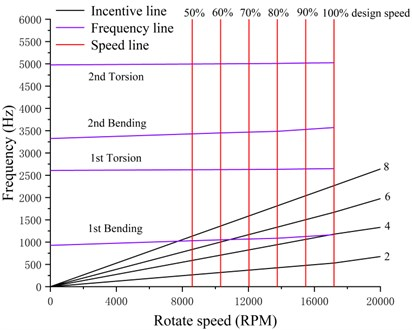## 5. Conclusions

Based on the CFD/CSD fluid-thermal-structure coupling method, this paper analyzes the influence of the aerodynamic load, thermal load and centrifugal load of the transonic axial flow compressor on the structural characteristics of the blade. The deformation and stress of the compressor blade in the case of solid coupling, and the influence of different loads on the structural characteristics of the compressor blade are analyzed. The blade is modal analysis under prestress, and the Campbell diagram of the blade is obtained. The main conclusions are obtained. as follows:

2) At different speeds, the deformation of the blades will first increase and then decrease with the continuous increase of the mass flow. The equivalent stress of the blades changes with the change of the mass flow and is related to the change of the speed. We are designing and Pay more attention when inspecting.

3) Considering the actual working conditions of the compressor, the natural frequency of the blade obtained by thermal load, centrifugal load and aerodynamic load has a huge change compared with no load. The first-order vibration frequency with the largest change increases by 25.77 %. Therefore, in the modal analysis of the blade, it is necessary to comprehensively consider the impact of various loads on the blade, and conduct a modal analysis based on fluid-thermal-structure coupling prestress.

4) The compressor blades have a resonance point at about 63 % and 98 % speed. In this case, the blades are prone to resonance, and more attention should be paid in the later analysis.

#### Acknowledgements

This work was supported by the Science Technology Department Key Project of Shaanxi Province (No. 2017ZDXM-GY-138), Key Project of Shaanxi Provincial Department of Science and Technology (No. 2019TSLGY02-03), General Project of Shaanxi Provincial Department of Science and Technology (No. 2020JM-600), and Shaanxi Provincial Department of Education Scientific Research Project (19JK0172).